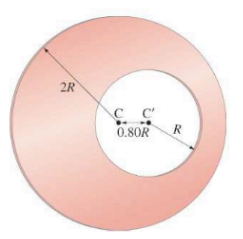×
Get Full Access to Physics: Principles With Applications - 6 Edition - Chapter 7 - Problem 52p
Get Full Access to Physics: Principles With Applications - 6 Edition - Chapter 7 - Problem 52p

×

# (III) A uniform circular plate of radius 2R has a circularISBN: 9780130606204 3

## Solution for problem 52P Chapter 7

Physics: Principles with Applications | 6th Edition

• Textbook Solutions
• 2901 Step-by-step solutions solved by professors and subject experts
• Get 24/7 help from StudySoup virtual teaching assistantsPhysics: Principles with Applications | 6th Edition

4 5 1 397 Reviews
27
1
Problem 52P

(III) A uniform circular plate of radiushas a circular hole of radiuscut out of it. The center $$C^{\prime}) of the smaller circle is a distance \(0.80 R$$ from the centerof the larger circle, Fig.. What is the position of the center of mass of the plate? [Hint: Try subtraction.]FIGURE 7-41 Problem 52

Equation Transcription:Text Transcription:

C'

0.80R

Step-by-Step Solution:
Step 1 of 6

We have to find the position of the center of mass of the resultant larger plate formed due to the removal of the small circular plate from it.

Step1 of 6

Concept:

Center of mass(CM) of a body is an imaginary point at which the whole mass of the body is supposed to be concentrated. We consider that the net force acts on the centre of mass of body, insteads of considering that it acts on each and every point on the body for simpler calculation and this is one of the importance of application of CM.

The location of the center of mass on the x-axis and y-axis is given asandStep 2 of 6

Step 3 of 6

##### ISBN: 9780130606204

Unlock Textbook Solution